# GSEB Solutions Class 8 Maths Chapter 3 Understanding Quadrilaterals Ex 3.1

Gujarat Board GSEB Textbook Solutions Class 8 Maths Chapter 3 Understanding Quadrilaterals Ex 3.1 Textbook Questions and Answers.

### Gujarat Board Textbook Solutions Class 8 Maths Chapter 3 Understanding Quadrilaterals Ex 3.1

Question 1.
Given here are some figures.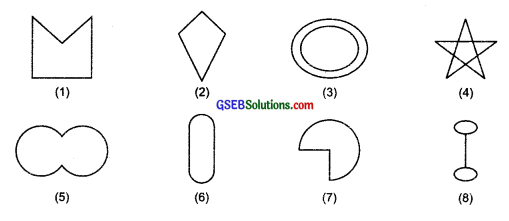Classify each of them on the basis of the following:
(a) Simple curve
(b) Simple closed curve
(c) Polygon
(d) Convex polygon
(e) Concave polygon
Solution:
(a) Simple curves are: (1), (2), (5), (6) and (7)
(b) Simple closed curves are: (1), (2), (5), (6) and (7).
(c) Polygons are: (1), (2) and (4).
(d) Convex polygon is: (2).
(e) Concave polygons are (1) and (4).Question 2.
How many diagonals does each of the following have?
(b) A regular hexagon
(c) A triangle
Solution:
Note: Number of diagonals in a polygon of n-sides = [$$\frac { n(n-1) }{ 2 }$$-n]
(a) In quadrilateral, number of sides (n) = 4
∴Number of diagonals = $$\frac { n(n-1) }{ 2 }$$ – n

Question 3.
What is the sum of the measures of the angles of a convex quadrilateral? Will this property hold if the quadrilateral is not convex? (Make a non-convex quadrilateral and try!)
Solution:
The sum of the measures of the angles of a convex quadrilateral = 360°
Yes, this property holds, even if the quadrilateral is not convex.

Question 4.
Examine the table. (Each figure is divided into triangle and the sum of the angles deduced from that)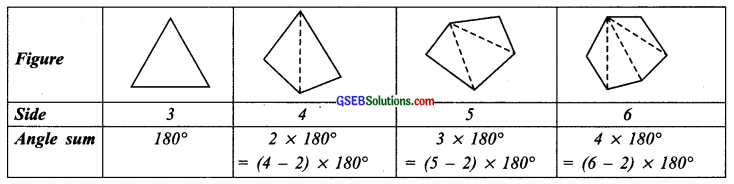What can you say about the angle sum of a convex polygon with number of sides?
(a) 7
(b) 8
(c) 10
(d) n
Solution:
From the above table, we conclude that sum of the interior angles of polygon with n-sides = (n – 2) x 180°
(a) When n = 7 Substituting n = 7 in the above formula, we have
Sum of interior angles of a polygon of 7 sides (i.e., when n = 7) = (n – 2) x 180° = (7 – 2) x 180° = 5 x 180° = 900°(b) When n = 8
Substituting n = 8 in the above formula, we have
Sum of interior angles of a polygon having 8 sides
= (u – 2) x 180° = (8 – 2) x 180°
= 6 x 180° = 1080°

(c) When n = 10
Substituting n = 10 in the above formula, we have
Sum of interior angles of a polygon having 10 sides
= (n – 2) x 180° = (10 – 2) x 180°
= 8 x 180° = 1440°

(d) When n = n
The sum of interior angles of a polygon having n-sides = (n – 2) x 180°

Question 5.
What is a regular polygon?
State the name of a regular polygon of
(i) 3 sides
(ii) 4 sides
(iii) 6 sides
Solution:
A polygon is said to be a regular polygon if
(a) The measures of its interior angles are equal, and .
(b) The lengths of its sides are equal.
The name of a regular polygon having
(i) 3 sides is ‘equilateral-triangle’.
(ii) 4 sides is ‘square’.
(iii) 6 sides is ‘regular hexagon’.Question 6.
Find the angle measure x in the following figures.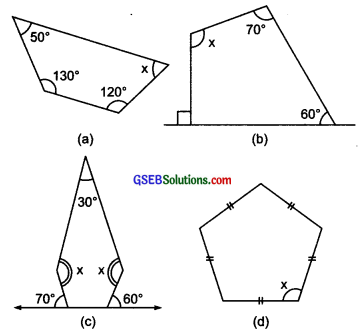Solution:
(a) v The sum of interior angles of a quadrilateral = 360°
x + 120° + 130° + 50° = 360°
or x + 300° = 360°
or x = 360° – 300° = 60°

(b) The sum of interior angles of a quadrilateral 360°
x + 60° + 70° + 90° = 360°
or
x + 220° = 360°
or
x = 360° – 220° = 140°(c) Interior angles are: 30°, x°, x°, (180° – 70°) and (180° – 60°),
i. e., 30°, x°, x°, 110° and 120°
The given figure is a pentagon,
∴ Sum of interior angles of a pentagon = 540°
∴30° + x + x + 110° + 120° = 540°
or 2x + 260° = 540°
or x = $$\frac { 540° – 260° }{ 2 }$$ = $$\frac { 280° }{ 2 }$$ = 140

(d) It is a regular pentagon.
Sum of all interior angles of a regular pentagon = 540°.
∴ Its each angle is equal.
∴ x + x + x + x + x= 540°
or 5x = 540°
or x = 540° ÷ 5 = 108°

Question 7.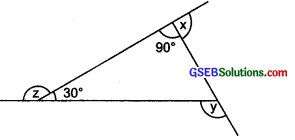(a) Find x + y + z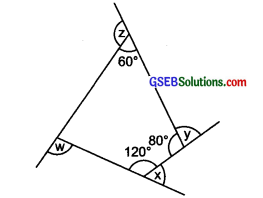(b) Find x + y + z + w
Solution:
(a) ∵ v x + 90° = 180° [Linear pair]
∴ x = 180° – 90° = 90°
y = 30° + 90° = 120°
[∴ Sum of interior opposite angles = exterior angle]
z = 180° – 30° = 150°
Now, x + y + z = 90° + 120° + 150° = 360°(b) ∵ The sum of interior angles of a quadrilateral = 360°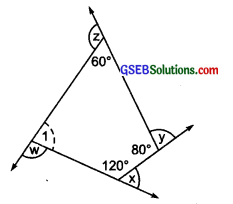∴∠1 + 120° + 80° + 60° = 360°
or ∠1 + 260° = 3600
or = ∠1 = 360° – 260° = 100°
Now, x + 120° 180° [Linear pair]
∴ x – 180° – 120° = 60°
y + 80° = 180° [Linear pair]
Y = 180° – 80° = 100°
z + 60° = 180° [Linear pair]
∴ z = 180° – 60° = 120°
w + 100° = 180° [Linear pair]
w = 180° – 100° = 80°
Thus, x + y + z + w
= 60° + 100° + 120° + 80° = 360°